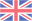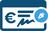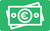# Ecole de Surf, Storm Surfing

## Stand Up Paddle ,  Surfing in Erquy

• Spoken languages
•Rates
Payment methods
••• Rates
• Base rate
• Base rate
• Base rate
• Base rate
• Base rate
• Base rate
• Base rate
• Base rate
• Base rate
• Base rate
• Base rate
• Base rate
• Base rate
• Base rate
• Base rate
• Base rate
• Base rate
• Base rate